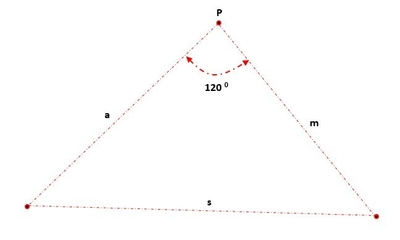# A plane is flying at a constant altitude at a speed of 600 \ mph. An anti-aircraft missile is...

## Question:

A plane is flying at a constant altitude at a speed of {eq}600 \ mph {/eq}. An anti-aircraft missile is fired and files at a speed of {eq}1200 \ mph {/eq}. The missile and plane approach the same point(call it "{eq}P {/eq}"); the angle between the paths of the plane and missile is {eq}120 ^\circ {/eq}.Determine how fast the distance between the plane and the missile is changing when the plane is {eq}100 {/eq} miles from {eq}P {/eq} and the missile is {eq}150 {/eq} miles from {eq}P {/eq}.

## Time Rates:

The problem is about time rates and to solve this we need to use the law of cosine which is {eq}c^{2}=a^{2}+b^{2}-2ab\cos C {/eq} where {eq}a,b,c {/eq} are the sides of the triangle and {eq}C {/eq} is the angle between the sides {eq}a {/eq} and {eq}b {/eq}.

## Answer and Explanation:

Below is the figure,From the figure above, using the law of cosine,

{eq}s^{2}=a^{2}+m^{2}-2am\cos 120 {/eq}

Differentiate the equation with respect to {eq}t {/eq}

{eq}\displaystyle 2s\frac{ds}{dt}=2a\frac{da}{dt}+2m\frac{dm}{dt}+\left ( a\frac{dm}{dt}+m\frac{da}{dt} \right ) {/eq}

Note that from the given {eq}a=100,\:\frac{da}{dt}=600,\:m=150,\:\frac{dm}{dt}=1200 {/eq}

When {eq}a=100,\:b=150,\:C=120 {/eq}

Then,

{eq}\displaystyle s^{2}=(100)^{2}+\left ( 150 \right )^{2}-2(100)(150)\cos 120^{0} {/eq}

{eq}s=50\sqrt{19} {/eq}

Thus,

{eq}\displaystyle 2(50\sqrt{19})\frac{ds}{dt}=2(100)(600)+2(150)(1200)+100(1200)+150(600) {/eq}

{eq}\displaystyle \frac{ds}{dt}\approx 3,165.94\:mph {/eq}

#### Learn more about this topic:Differential Calculus: Definition & Applications

from Calculus: Help and Review

Chapter 13 / Lesson 6
18K